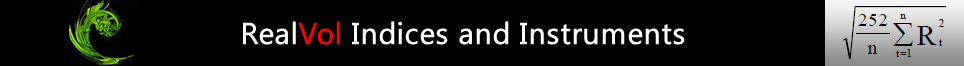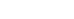# RealVol Daily Formula(Realized Volatility Formulas)

The RealVol daily formula is used for calculation of the realized volatility indices and realized volatility of volatility indices (RVOL and RVOV).  Those indices are used for contract settlement (expiration) of RealVol instruments.

### Design of RealVol Daily FormulaMean Set to Zero
The RealVol Daily Formula starts with the traditional formula for standard deviation and modifies it in a few key ways. First, we set the mean to zero in order to provide “movement regardless of direction” instead of “movement about a mean or trend.” Doing so makes hedging easier for options traders and corresponds to the formula used for variance swaps and volatility swaps in the over-the-counter market.

Annualization Factor
Second, RealVol sets the annualization factor to a constant. The constant value of 252 represents the number of trading days in a typical year in the U.S. Because of the vagaries of the calendar in any particular year and/or the holiday schedules in any particular country, the actual number of trading days may be slightly higher or lower than 252. However, it is preferable to have one approximate constant than to have a variety of exact, but different, values.

Degrees of Freedom
Third, “degrees of freedom” is a term in statistics used to extrapolate from a sample of data to the entire dataset. Since the intent is to provide the exact realized volatility over a specific period and not to extrapolate that sample dataset to the entire history of trading, RealVol sets the degrees of freedom to zero.

Dollar and Cents Construct
Finally, the result is typically a value less than 1.00. RealVol multiplies the result by 100 in order to bring the values to a more intuitive “dollars and cents” construct. For example, the annualized realized volatility of an equity index may be 0.20. Often, traders would quote this number as 20%. RealVol would disseminate the index value as 20.00.

### Formula 1Where:

Vol = Realized volatility
252 = a constant representing the approximate number of trading days in a year
t = a counter representing each trading day
n = number of trading days in the measurement time frame
Rt = continuously compounded daily returns as calculated by the formula:

### Formula 2Where:

Ln = natural logarithm
Pt = Underlying Reference Price (“closing price”) at day t
Pt–1 = Underlying Reference Price at day immediately preceding day t

Also see RealVol Real-Time Formula.